# High School Physics : Waves, Sound, and Light

## Example Questions

← Previous 1 3 4 5 6 7 8 9

### Example Question #1 : Waves, Sound, And Light

Two waves, each with an amplitude of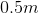are superimposed with constructive interference such that they are in phase. What is the resultant amplitude?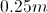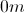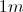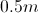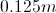Explanation:

When two waves are superimposed, the interference can either be constructive or destructive. In this case, the interference is constructive and the waves are in phase, which means we add the amplitudes together.

Since each wave has an amplitude of, our new amplitude will be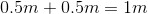.

### Example Question #1 : Waves, Sound, And Light

Two waves, one with an amplitude of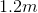and the other of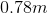are superimposed with destructive interference. What is the resultant amplitude?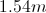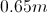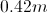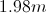Explanation:

When two waves are superimposed, the interference can be either constructive or destructive. In this case the interference is destructive, which means our resultant amplitude will be the difference of the two given amplitudes.

That means our new amplitude will be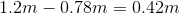.

### Example Question #1 : Understanding The Concept Of Waves

Two identical waves are superimposed with destructive interference. What will happen to the resulting wave?

It will be twice as large as each original wave.

It will be half as large as each original wave.

It will be still. There will be no wave.

The two waves will pass through each other without problem.

It will be still. There will be no wave.

Explanation:

When two waves are superimposed with destructive interference, they cancel each other out. Since these two waves are identical, they will completely cancel each other out and there will be no wave. It will be still.

### Example Question #4 : Waves, Sound, And Light

Order the following electromagnetic waves from the longest wavelength to the shortest: Gamma Rays, Infrared, Microwaves, Radio Waves, Ultraviolet, Visible Light, X-Rays

Visible Light, Infrared, Microwaves, Gamma Rays, X-Rays, Ultraviolet, Radio Waves

Gamma Rays, Infrared, Microwaves, Radio Waves, Ultraviolet, Visible Light, X-Rays

Radio Waves, Microwaves, Infrared, Visible Light, Ultraviolet, X-Rays, Gamma Rays

Gamma Rays, X-Rays, Ultraviolet, Visible Light, Infrared, Microwaves, Radio Waves

Radio Waves, Gamma Rays, Microwaves, Infrared, Visible Light, X-Rays, Ultraviolet

Radio Waves, Microwaves, Infrared, Visible Light, Ultraviolet, X-Rays, Gamma Rays

Explanation:

Radio waves have the smallest frequency and longest wavelength. This is why they are not dangerous. Microwaves have the next longest wavelength and are what are used to warm up cold foods. Infrared waves are the next longest wavelength and border the red light on the visible spectrum. Infrared waves are used in remote controls and night vision. Visible light is next and is what we can see with our eyes. Next is ultraviolet, which borders the violet light on the visible spectrum. These are the damaging rays by the sun and are essentially “super-violet” rays. Next is X-rays. These have very high frequencies and are dangerous in high quantities. Gamma rays are the shortest wavelength and the highest frequency and the most dangerous. These cosmic rays often come from stars and other celestial objects.

### Example Question #5 : Waves, Sound, And Light

Two waves are traveling toward each other along a rope. When they meet, the waves

disappear

pass through each other

bounce off each other

pass through each other

Explanation:

When waves interfere with one another, they pass through each, and others can undergo either constructive or destructive interference.  In constructive interference, waves add together to produce a briefly more massive wave.  In destructive interference, waves subtract from each other to create a smaller wave.  However, once the waves move past one another, they will return to their original shape and size.

### Example Question #6 : Waves, Sound, And Light

A wave transports

matter but not energy

both energy and matter

energy but not matter

energy but not matter

Explanation:

Waves carry energy along the path displacing the matter for a brief period. However, the matter does not travel along the wave and instead will return to its natural rest position once the wave moves past it. For example, an ocean wave can travel many miles without displacing the entire ocean.

### Example Question #7 : Waves, Sound, And Light

In seismology, the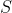wave is a transverse wave. As anwave travels through the Earth, the relative motion between thewave and the particles is

First parallel, then perpendicular

First perpendicular, then parallel

Parallel

Perpendicular

Perpendicular

Explanation:

Transverse waves are waves whose particles travel perpendicular to the direction that the wave itself is traveling. Electromagnetic waves are another example of transverse waves.

### Example Question #8 : Waves, Sound, And Light

A radio station broadcasts at a frequency of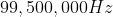. If the broadcast is an electromagnetic wave, then what is its wavelength?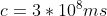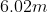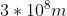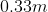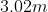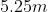Explanation:

The relationship between wavelength and frequency is given by the equation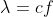where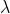is the wavelength,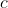is the speed of light, and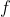is frequency.

We are given the values for frequency and the speed of light, allowing us to solve for the wavelength.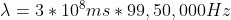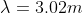### Example Question #9 : Waves, Sound, And Light

In a vacuum, the velocity of all electromagnetic waves

is nearly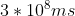depends on the frequency

depends on the amplitude

Is zero

is nearlyExplanation:

Electromagnetic waves all travel at the same speed which is the speed of light.  The speed of light in a vacuum is nearly### Example Question #10 : Waves, Sound, And Light

Which of the following waves require a medium to travel?

Sound Waves

Light Waves

Microwaves